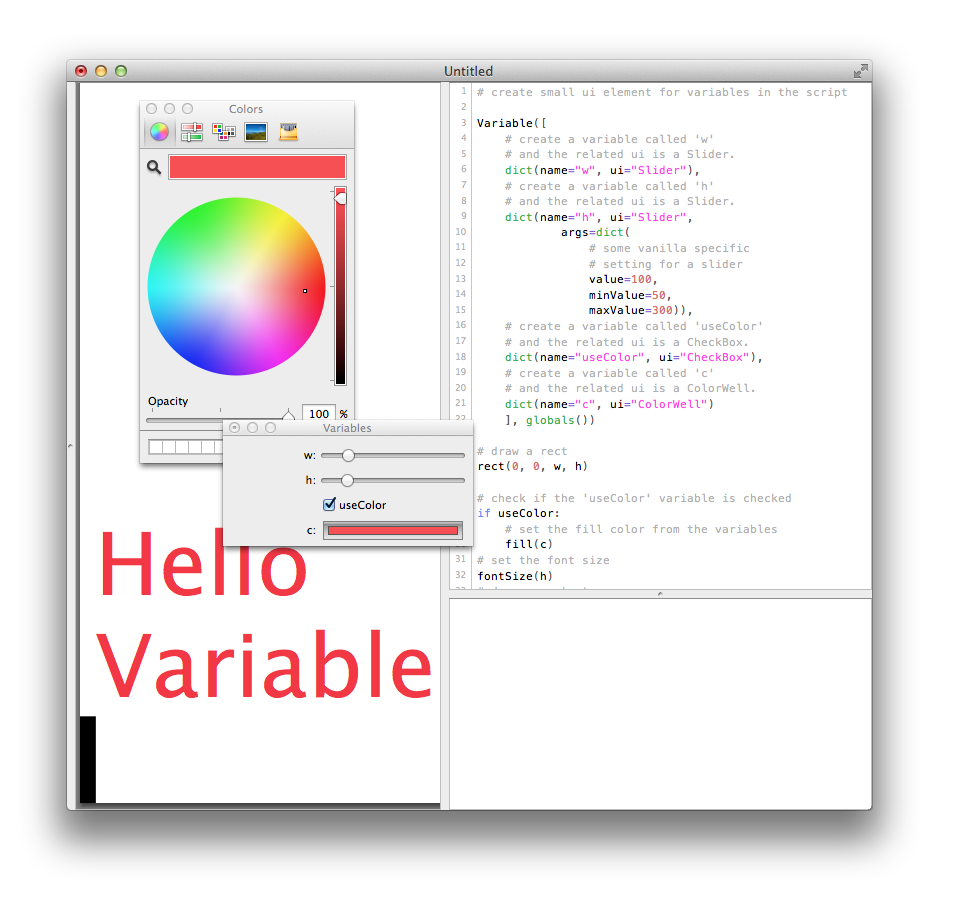# Variables¶

`Variable`(variables, workSpace)

Build small UI for variables in a script.

The workSpace is usually globals() as you want to insert the variable in the current workspace. It is required that workSpace is a dict object.```# create small ui element for variables in the script

Variable([
# create a variable called 'w'
# and the related ui is a Slider.
dict(name="w", ui="Slider"),
# create a variable called 'h'
# and the related ui is a Slider.
dict(name="h", ui="Slider",
args=dict(
# some vanilla specific
# setting for a slider
value=100,
minValue=50,
maxValue=300)),
# create a variable called 'useColor'
# and the related ui is a CheckBox.
dict(name="useColor", ui="CheckBox"),
# create a variable called 'c'
# and the related ui is a ColorWell.
dict(name="c", ui="ColorWell")
], globals())

# draw a rect
rect(0, 0, w, h)

# check if the 'useColor' variable is checked
if useColor:
# set the fill color from the variables
fill(c)
# set the font size
fontSize(h)
# draw some text
text("Hello Variable", (w, h))
``````# Variable == vanilla power in DrawBot
from AppKit import NSColor
# create a color
_color = NSColor.colorWithCalibratedRed_green_blue_alpha_(0, .5, 1, .8)
# setup variables using different vanilla ui elements.
Variable([
dict(name="aList", ui="PopUpButton", args=dict(items=['a', 'b', 'c', 'd'])),
dict(name="aText", ui="EditText", args=dict(text='hello world')),
dict(name="aSlider", ui="Slider", args=dict(value=100, minValue=50, maxValue=300)),
dict(name="aCheckBox", ui="CheckBox", args=dict(value=True)),
dict(name="aColorWell", ui="ColorWell", args=dict(color=_color)),